The COUNTREG Procedure

OUTPUT Statement

• OUTPUT <OUT=SAS-data-set> <output-options> ;

The OUTPUT statement creates a new SAS data set that contains all the variables in the input data set and, optionally, the estimates of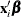, the expected value of the response variable, and the probability of the response variable taking on the current value or other values that you specify. In a zero-inflated model, you can additionally request that the output data set contain the estimates of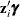and the probability that the response is zero as a result of the zero-generating process. For the Conway-Maxwell-Poisson model, the estimates of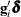,,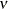,, mode, variance, and dispersion are also available. Except for the probability of the current value, these statistics can be computed for all observations in which the regressors are not missing, even if the response is missing. By adding observations that have missing response values to the input data set, you can compute these statistics for new observations or for settings of the regressors that are not present in the data without affecting the model fit.

You can specify only one OUTPUT statement. You can specify the following output-options:

OUT=SAS-data-set

names the output data set.

XBETA=name

names the variable that contains estimates of.

PRED=name
MEAN=name

assigns a name to the variable that contains the predicted value of the response variable.

PROB=name

names the variable that contains the probability of the response variable taking the current value, Pr(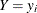).

PROBCOUNT(value1 <value2...>)

outputs the probability that the response variable will take particular values. Each value should be a nonnegative integer. Nonintegers are rounded to the nearest integer. The value can also be a list of the form X TO Y BY Z. For example, PROBCOUNT(0 1 2 TO 10 BY 2 15) requests predicted probabilities for counts 0, 1, 2, 4, 5, 6, 8, 10, and 15. This option is not available for the fixed-effects and random-effects panel models.

ZGAMMA=name

names the variable that contains estimates of.

PROBZERO=name

names the variable that contains the value of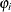, the probability of the response variable taking on the value of zero as a result of the zero-generating process. It is written to the output file only if the model is zero-inflated. This is not the overall probability of a zero response; that is provided by the PROBCOUNT(0) option.

GDELTA=name

assigns a name to the variable that contains estimates offor the Conway-Maxwell-Poisson distribution.

LAMBDA=name

assigns a name to the variable that contains the estimate offor the Conway-Maxwell-Poisson distribution.

NU=name

assigns a name to the variable that contains the estimate offor the Conway-Maxwell-Poisson distribution.

MU=name

assigns a name to the variable that contains the estimate offor the Conway-Maxwell-Poisson distribution.

MODE=name

assigns a name to the variable that contains the integral part of(mode) for the Conway-Maxwell-Poisson distribution.

VARIANCE=name

assigns a name to the variable that contains the estimate of variance for the Conway-Maxwell-Poisson distribution.

DISPERSION=name

assigns a name to the variable that contains the value of dispersion for the Conway-Maxwell-Poisson distribution.